Algebra Commons™

1,064 Full-Text Articles 1,179 Authors 224,226 Downloads116 Institutions

All Articles in Algebra

1,064 full-text articles. Page 1 of 40.

Patterns, Symmetries, And Mathematical Structures In The Arts, 2020Georgia Southern University

Patterns, Symmetries, And Mathematical Structures In The Arts, Sarah C. Deloach

University Honors Program Theses

Mathematics is a discipline of academia that can be found everywhere in the world around us. Mathematicians and scientists are not the only people who need to be proficient in numbers. Those involved in social sciences and even the arts can benefit from a background in math. In fact, connections between mathematics and various forms of art have been discovered since as early as the fourth century BC. In this thesis we will study such connections and related concepts in mathematics, dances, and music.

2019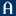Augustana College, Rock Island Illinois

The Last Digits Of Infinity (On Tetrations Under Modular Rings), William Stowe

Celebration of Learning

A tetration is defined as repeated exponentiation. As an example, 2 tetrated 4 times is 2^(2^(2^2)) = 2^16. Tetrated numbers grow rapidly; however, we will see that when tetrating where computations are performed mod n for some positive integer n, there is convergent behavior. We will show that, in general, this convergent behavior will always show up.

Dehn Functions Of Bestvina-Brady Groups, 2019Louisiana State University and Agricultural and Mechanical College

Dehn Functions Of Bestvina-Brady Groups, Yu-Chan Chang

LSU Doctoral Dissertations

In this dissertation, we prove that if the flag complex on a finite simplicial graph is a 2-dimensional triangulated disk, then the Dehn function of the associated Bestvina--Brady group depends on the maximal dimension of the simplices in the interior of the flag complex. We also give some examples where the flag complex on a finite simplicial graph is not 2-dimensional, and we establish a lower bound for the Dehn function of the associated Bestvina--Brady group.

2019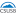California State University - San Bernardino

Analogues Between Leibniz's Harmonic Triangle And Pascal's Arithmetic Triangle, Lacey Taylor James

Electronic Theses, Projects, and Dissertations

This paper will discuss the analogues between Leibniz's Harmonic Triangle and Pascal's Arithmetic Triangle by utilizing mathematical proving techniques like partial sums, committees, telescoping, mathematical induction and applying George Polya's perspective. The topics presented in this paper will show that Pascal's triangle and Leibniz's triangle both have hockey stick type patterns, patterns of sums within shapes, and have the natural numbers, triangular numbers, tetrahedral numbers, and pentatope numbers hidden within. In addition, this paper will show how Pascal's Arithmetic Triangle can be used to construct Leibniz's Harmonic Triangle and show how both triangles ...

Cone-Constrained Rational Eigenvalue Problems, 2019University of Avignon

Cone-Constrained Rational Eigenvalue Problems, Alberto Seeger

Electronic Journal of Linear Algebra

This work deals with the eigenvalue analysis of a rational matrix-valued function subject to complementarity constraints induced by a polyhedral cone $K$. The eigenvalue problem under consideration has the general structure $\left(\sum_{k=0}^d \lambda^k A_k + \sum_{k =1}^m \frac{p_k(\lambda)}{q_k(\lambda)} \,B_k\right) x = y , \quad K\ni x \perp y\in K^\ast,$ where $K^\ast$ denotes the dual cone of $K$. The unconstrained version of this problem has been discussed in [Y.F. Su and Z.J. Bai. Solving rational eigenvalue problems via linearization. \emph{SIAM J. Matrix Anal. Appl.}, 32 ...

Minimum Rank, Maximum Nullity, And Zero Forcing Number Of Graphs, 2019University of Regina

Minimum Rank, Maximum Nullity, And Zero Forcing Number Of Graphs, Shaun M. Fallat, Leslie Hogben

Leslie Hogben

This chapter represents an overview of research related to a notion of the “rank of a graph" and the dual concept known as the “nullity of a graph," from the point of view of associating a fixed collection of symmetric or Hermitian matrices to a given graph. This topic and related questions have enjoyed a fairly large history within discrete mathematics, and have become very popular among linear algebraists recently, partly based on its connection to certain inverse eigenvalue problems, but also because of the many interesting applications (e.g., to communication complexity in computer science and to control of ...

Pairwise Completely Positive Matrices And Conjugate Local Diagonal Unitary Invariant Quantum States, 2019Mount Allison University

Pairwise Completely Positive Matrices And Conjugate Local Diagonal Unitary Invariant Quantum States, Nathaniel Johnston, Olivia Maclean

Electronic Journal of Linear Algebra

A generalization of the set of completely positive matrices called pairwise completely positive (PCP) matrices is introduced. These are pairs of matrices that share a joint decomposition so that one of them is necessarily positive semidefinite while the other one is necessarily entrywise non-negative. Basic properties of these matrix pairs are explored and several testable necessary and sufficient conditions are developed to help determine whether or not a pair is PCP. A connection with quantum entanglement is established by showing that determining whether or not a pair of matrices is pairwise completely positive is equivalent to determining whether or not ...

On The Intersection Number Of Finite Groups, 2019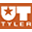University of Texas at Tyler

On The Intersection Number Of Finite Groups, Humberto Bautista Serrano

Math Theses

Let G be a finite, nontrivial group. In a paper in 1994, Cohn defined the covering number of a finite group as the minimum number of nontrivial proper subgroups whose union is equal to the whole group. This concept has received considerable attention lately, mainly due to the importance of recent discoveries. In this thesis we study a dual concept to the covering number. We define the intersection number of a finite group as the minimum number of maximal subgroups whose intersection is equal to the Frattini subgroup. Similarly we define the inconjugate intersection number of a finite group as ...

2019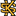Kennesaw State University

The Effect Of Experiential Learning On Students’ Conceptual Understanding Of Functions In Algebra 1, Jeremiah Veillon

Doctor of Education in Secondary Education Dissertations

For years, traditional mathematics instruction has prioritized memory over thought; this often leads to a disconnect between mathematics and real life, which is a contributing factor for the increased rate of college students having to take remedial mathematics. Experiential Learning (EL) seeks to influence students’ decisions to transfer what they are learning to the classroom by engaging students’ emotional processes through concrete experiences. EL has been successful over the years in increasing student engagement and conceptual understanding; however, more research is needed to examine the effect of EL with secondary mathematics students. The purpose of this study was to examine ...

Proof Of A Conjecture Of Graham And Lovasz Concerning Unimodality Of Coefficients Of The Distance Characteristic Polynomial Of A Tree, 2019Iowa State University

Proof Of A Conjecture Of Graham And Lovasz Concerning Unimodality Of Coefficients Of The Distance Characteristic Polynomial Of A Tree, Ghodratollah Aalipour, Aida Abiad, Zhanar Berikkyzy, Leslie Hogben, Franklin H.J. Kenter, Jephian C.-H. Lin, Michael Tait

Leslie Hogben

The conjecture of Graham and Lov ́asz that the (normalized) coefficients of the distance characteristic polynomial of a tree are unimodal is proved; it is also shown that the (normalized) coefficients are log-concave. Upper and lower bounds on the location of the peak are established.

2019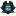East Tennessee State University

Taking Notes: Generating Twelve-Tone Music With Mathematics, Nathan Molder

Electronic Theses and Dissertations

There has often been a connection between music and mathematics. The world of musical composition is full of combinations of orderings of diﬀerent musical notes, each of which has diﬀerent sound quality, length, and em phasis. One of the more intricate composition styles is twelve-tone music, where twelve unique notes (up to octave isomorphism) must be used before they can be repeated. In this thesis, we aim to show multiple ways in which mathematics can be used directly to compose twelve-tone musical scores.

2019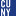The Graduate Center, City University of New York

On The Complexity Of Computing Galois Groups Of Differential Equations, Mengxiao Sun

All Dissertations, Theses, and Capstone Projects

The differential Galois group is an analogue for a linear differential equation of the classical Galois group for a polynomial equation. An important application of the differential Galois group is that a linear differential equation can be solved by integrals, exponentials and algebraic functions if and only if the connected component of its differential Galois group is solvable. Computing the differential Galois groups would help us determine the existence of the solutions expressed in terms of elementary functions (integrals, exponentials and algebraic functions) and understand the algebraic relations among the solutions.

Hrushovski first proposed an algorithm for computing the differential ...

2019The Graduate Center, City University of New York

A Differential Algebra Approach To Commuting Polynomial Vector Fields And To Parameter Identifiability In Ode Models, Peter Thompson

All Dissertations, Theses, and Capstone Projects

In the first part, we study the problem of characterizing polynomial vector fields that commute with a given polynomial vector field. One motivating factor is that we can write down solution formulas for an ODE that corresponds to a planar vector field that possesses a linearly independent commuting vector field. This problem is also central to the question of linearizability of vector fields. We first show that a linear vector field admits a full complement of commuting vector fields. Then we study a type of planar vector field for which there exists an upper bound on the degree of a ...

2019The Graduate Center, City University of New York

Analysis Of A Group Of Automorphisms Of A Free Group As A Platform For Conjugacy-Based Group Cryptography, Pavel Shostak

All Dissertations, Theses, and Capstone Projects

Let F be a finitely generated free group and Aut(F) its group of automorphisms.

In this monograph we discuss potential uses of Aut(F) in group-based cryptography.

Our main focus is on using Aut(F) as a platform group for the Anshel-Anshel-Goldfeld protocol, Ko-Lee protocol, and other protocols based on different versions of the conjugacy search problem or decomposition problem, such as Shpilrain-Ushakov protocol.

We attack the Anshel-Anshel-Goldfeld and Ko-Lee protocols by adapting the existing types of the length-based attack to the specifics of Aut(F). We also present our own version of the length-based attack that significantly increases ...

Understanding The Impact Of Peer-Led Workshops On Student Learning, 2019CUNY New York City College of Technology

Understanding The Impact Of Peer-Led Workshops On Student Learning, Afolabi Ibitoye, Armando Cosme, Nadia Kennedy

Publications and Research

As students we often wonder why some subjects are easy to understand and requires not much effort in terms of re-reading the material, for us to grasp what it entails. One subject seems to remain elusive and uneasy for a vast majority of learners at all levels of education; that subject is Mathematics, it is one subject that most learners finds difficult even after doubling the amount of time spent on studying the material. My intention is to explore ways to make Mathematics easier for other students using feedback from students enrolled in NSF mathematics peer leading workshops, and use ...

Weyl Groups And The Nil-Hecke Algebra, 2019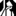University at Albany, State University of New York

Weyl Groups And The Nil-Hecke Algebra, Arta Holaj

Mathematics and Statistics

We begin this paper with a short survey on finite reflection groups. First we establish what a reflection in Euclidean space is. Then we introduce a root system, which is then partitioned into two sets: one of positive roots and one with negative roots. Th is articulates our understanding of groups generated by simple reflections. Furthermore, we develop our insight to Weyl groups and crystallographic groups before exploring crystallographic root systems. The section section of this paper examines the twisted group algebra along with the Demazure element Xi and the Demazure-Lusztig element Ti. Lastly, the third section of this paper ...

2019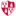Montclair State University

One Teacher's Transformation Of Practice Through The Development Of Covariational Thinking And Reasoning In Algebra : A Self-Study, Jacqueline Dauplaise

Theses, Dissertations and Culminating Projects

CCSSM (2010) describes quantitative reasoning as expertise that mathematics educators should seek to develop in their students. Researchers must then understand how to develop covariational reasoning. The problem is that researchers draw from students’ dialogue as the data for understanding quantitative relationships. As a result, the researcher can only conceive the students’ reasoning. The objective of using the self-study research methodology is to examine and improve existing teaching practices. To improve my practice, I reflected upon the implementation of my algebra curriculum through a hermeneutics cycle of my personal history and living educational theory. The critical friend provoked through dialogues ...

Integrating Mathematics And Educational Robotics: Simple Motion Planning, 2019Loyola University Chicago

Integrating Mathematics And Educational Robotics: Simple Motion Planning, Ronald I. Greenberg, George K. Thiruvathukal, Sara T. Greenberg

George K. Thiruvathukal

This paper shows how students can be guided to integrate elementary mathematical analyses with motion planning for typical educational robots. Rather than using calculus as in comprehensive works on motion planning, we show students can achieve interesting results using just simple linear regression tools and trigonometric analyses. Experiments with one robotics platform show that use of these tools can lead to passable navigation through dead reckoning even if students have limited experience with use of sensors, programming, and mathematics.

Vector Spaces Of Generalized Linearizations For Rectangular Matrix Polynomials, 2019Indian Institute of Technology Guwahati

Vector Spaces Of Generalized Linearizations For Rectangular Matrix Polynomials, Biswajit Das, Shreemayee Bora

Electronic Journal of Linear Algebra

The complete eigenvalue problem associated with a rectangular matrix polynomial is typically solved via the technique of linearization. This work introduces the concept of generalized linearizations of rectangular matrix polynomials. For a given rectangular matrix polynomial, it also proposes vector spaces of rectangular matrix pencils with the property that almost every pencil is a generalized linearization of the matrix polynomial which can then be used to solve the complete eigenvalue problem associated with the polynomial. The properties of these vector spaces are similar to those introduced in the literature for square matrix polynomials and in fact coincide with them when ...

Potentially Eventually Positive 2-Generalized Star Sign Patterns, 2019Huaiyin Institute of Technology

Potentially Eventually Positive 2-Generalized Star Sign Patterns, Yu Ber-Lin, Ting-Zhu Huang, Xu Sanzhang

Electronic Journal of Linear Algebra

A sign pattern is a matrix whose entries belong to the set $\{+, -, 0\}$. An $n$-by-$n$ sign pattern $\mathcal{A}$ is said to be potentially eventually positive if there exists at least one real matrix $A$ with the same sign pattern as $\mathcal{A}$ and a positive integer $k_{0}$ such that $A^{k}>0$ for all $k\geq k_{0}$. An $n$-by-$n$ sign pattern $\mathcal{A}$ is said to be potentially eventually exponentially positive if there exists at least one real matrix $A$ with the same sign pattern as $\mathcal{A}$ and a nonnegative integer \$t_ ...